# Pedro Tavares, Paulo Tabuada, ‡

 where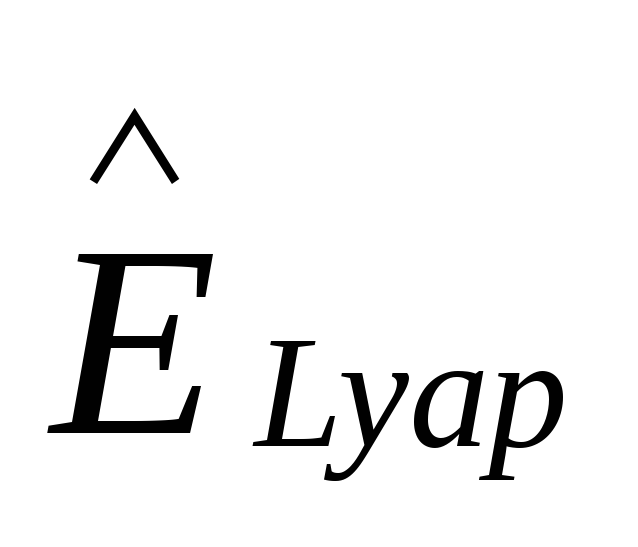is the Lyapunov candidate function computed using the predicted angular velocity. Assuming that the minimization algorithm is working correctly, we will have: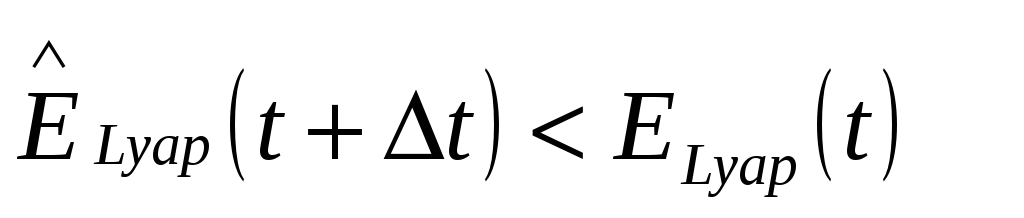(15) substituting (14) in (15) we get: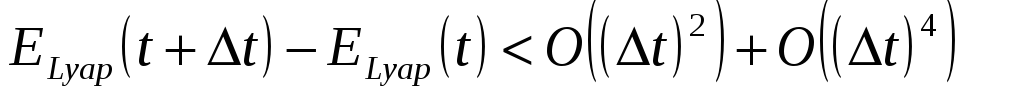(16) dividing by t and assuming t as small as wanted, we can write: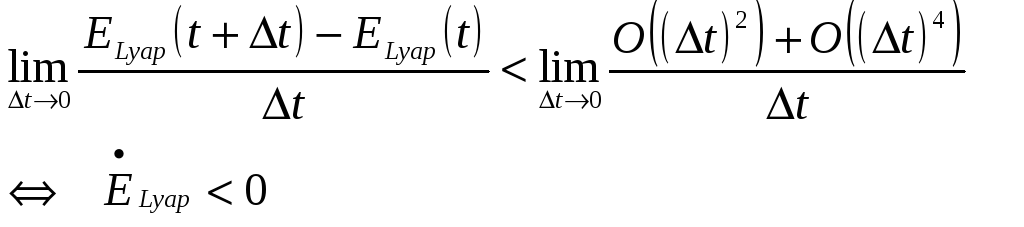(17) Therefore global uniform asymptotical stability is ensured towards the reference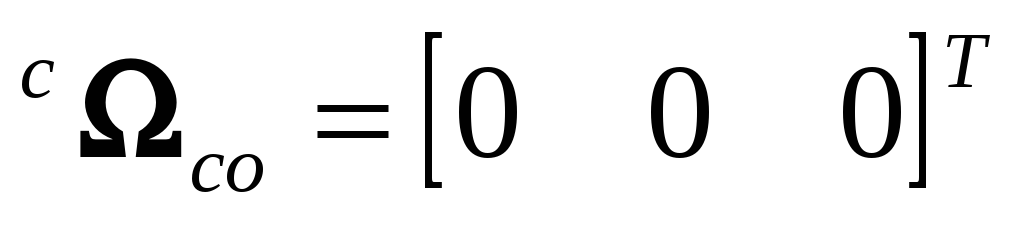, and as previously shown also towards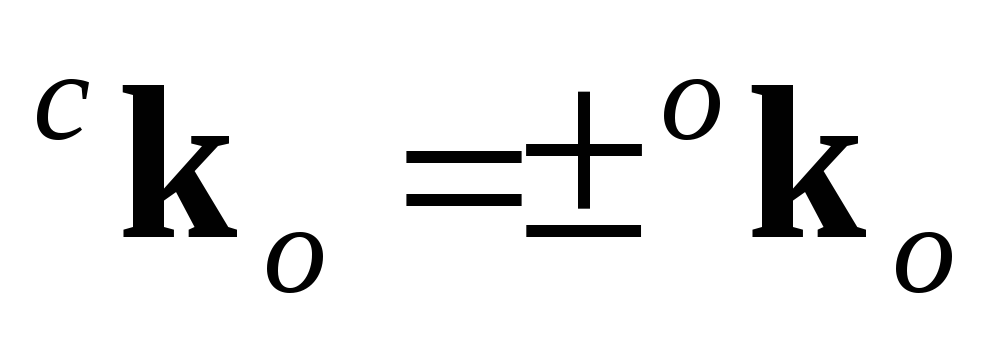. Implementation Unrestricted actuators For ideal actuators the minimization of the cost function is done on a continuous unlimited subset of a plan. An iterative method for the cost function minimization was required, so a Genetic Algorithm (GA) (Goldberg, 1989) was implemented. Any other iterative algorithm could be used but the GA was chosen because of its fast convergence characteristics, since in this problem the geomagnetic field is constantly changing. The implemented GA uses the standard techniques and two special operators: elitism, under which the best solution is always preserved and transmitted to the next generation and cloning, by which we insert into the population the solution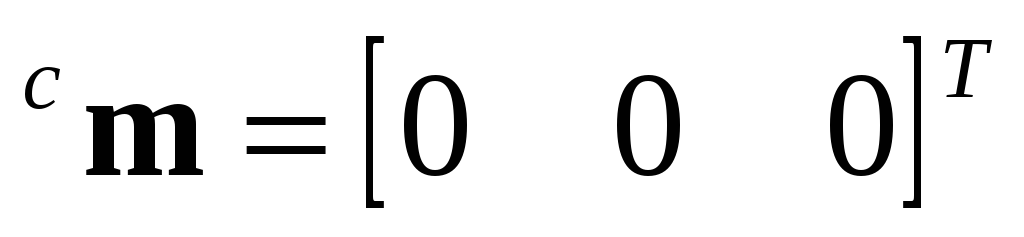. Cloning is justified because it has been found through simulation that sometimes the algorithm would converge to magnetic moments parallel to the geomagnetic field after the stabilization had been completed. The solution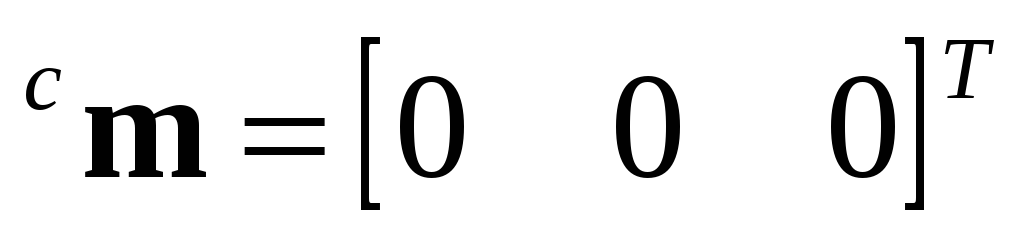performs same action (do nothing), but preserves power, as it does not use the magnetorquers for that purpose. Restricted actuators PoSAT-1 as other satellites of the UoSAT class has reduced control capabilities due to the restricted nature of its actuators. Satellite design factors have restricted the values of the control magnetic moment to only three different values of positive/negative polarity. Combining this restriction with the single-coil-actuation the available set of magnetic moments is reduced to only 18 different values (6 for the i coils, 6 for the j coils and 6 for the k coils). Power consumption is another serious restriction, which reflects on PoSAT actuation capabilities. For each actuation on a coil there must be at least a back-off time of 100 sec. to recharge the power supplies. This means that the actuators have at most a duty cycle of 3%, since the maximum actuation time is only 3 seconds. Considering these restraints, there are only 19 available magnetic moments: the 18 already referred and the do nothing solution. With such a restricted search space it is not necessary to use an iterative minimization algorithm, because all solutions may be evaluated and the best one (the one that minimizes (5)) is chosen. Simulation Results Several simulations were performed using the ConSat simulator, where perfect attitude determination is assumed and no disturbance torques (ex: aerodynamic drag or other effects) are considered. Simulations were performed for attitude stabilisation only and attitude stabilisation with spin control, where the cost function used was a variation of (5).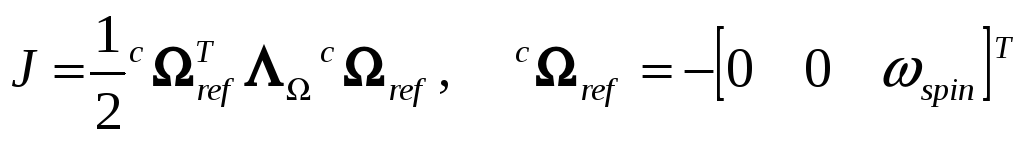(18) Figure 6 shows that the performance attained with the predictive algorithm, for attitude stabilisation only, is similar to energy based control proposed by (Wisniewski, 1997). , the angle between the local vertical and the boom axis, is reduced from 60º to less then 5º in only 3 orbits. It is interesting to note that the results attained with restricted actuators are similar to unrestricted actuators, and since the computational effort involved is considerably small this algorithm is valid solution for the on board computer resources. For attitude stabilisation and spin control the algorithm’s libration damping performance is slightly reduced since it is now necessary 4 orbits to reduce  to 5º and a steady state error of 2º is attained, while maintaining the spin velocity at a reference of 0.02 rad/s. Figure 7 shows that spin velocity oscillates around the reference while libration is being dampened but the set point is attained again as soon as the perturbation is rejected and the oscillation amplitudes reduced, being smaller than 0.0006 rad/s. To test the spin control algorithm performance the satellite was spinned-up from 0 to 0.02 rad/s with an initial  value of 5º. Simulation results show that the predictive algorithm takes less than 19.2 minutes or 12 actuations to set the spin velocity within a neighbourhood of 0.001 rad/s and achieves a final accuracy of less than 0.0005 rad/s. These are encouraging results since the actuators’ restrictions are quite severe. However, the dissipated energy is superior to energy based control since it is impossible to generate a magnetic moment perpendicular to the geomagnetic field at all actuation instants.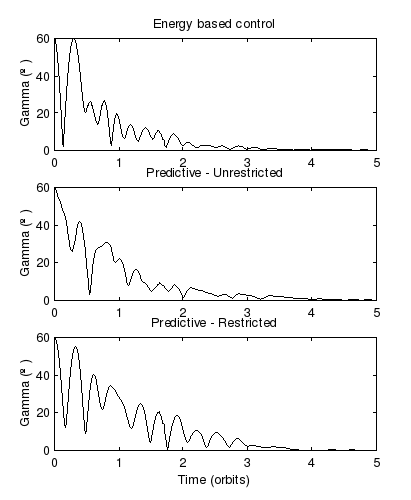Download 1.52 Mb.Share with your friends: